## Example Questions

### Example Question #21 : How To Find Data Representation In Physics

A scientist is testing the efficiency of a regular heat engine. A heat engine functions in the following manner: a chamber of gas is heated, increasing its pressure, which then causes the gas to expand to relieve some of that pressure. Next, the gas chamber cools off slightly, causing the pressure to decrease once again. This propagates the gas to compress slightly. Simply stated, this process produces mechanical work as the gas's expansion and contraction causes movement. A graph of the heat engine's pressure and volume is represented below, the letters A through D representing steps in the engine's repeating cycle.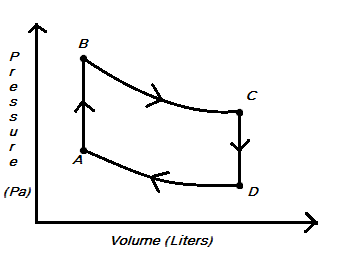Mechanical work for a gas process (movement from one pressure-volume state to another) is calculated using the formula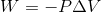, where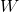represents work,represents pressure (which may or may not be constant), and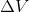represents a change in volume.

A gas process is considered isochoric if the volume is held constant throughout the process. Which of the gas processes shown above can be considered isochoric?

C to D

A to B and C to D

D to A

B to C

A to B

A to B and C to D

Explanation:

If we look at any gas process involved in this cycle, the volume only appears to change from A to B and from C to D. This we know because when we trace the cycle, it is only in these processes that we are not moving at all along the-axis.

### Example Question #1331 : Act Science

A scientist is testing the efficiency of a regular heat engine. A heat engine functions in the following manner: a chamber of gas is heated, increasing its pressure, which then causes the gas to expand to relieve some of that pressure. Next, the gas chamber cools off slightly, causing the pressure to decrease once again. This propagates the gas to compress slightly. Simply stated, this process produces mechanical work as the gas's expansion and contraction causes movement. A graph of the heat engine's pressure and volume is represented below, the letters A through D representing steps in the engine's repeating cycle.Mechanical work for a gas process (movement from one pressure-volume state to another) is calculated using the formula, whererepresents work,represents pressure (which may or may not be constant), andrepresents a change in volume.

The temperature of a gas is positively correlated with the pressure of that gas and negatively correlated with the volume of that gas. At which point is the gas in the engine's chamber at a maximum temperature?

Point A

Point C

The gas never reaches a maximum temperature.

Point B

Point D

Point B

Explanation:

This question may seem like it is asking for extraneous information, but in reality, it is just asking you to interpret the diagram given. If the temperature is positively correlated with pressure and negatively correlated with volume, we are looking for a point with the highest pressure and lowest volume. At the lowest volume are points A and B. Point B is at a higher pressure, indicating that it likely has a higher temperature than point A. Therefore, Point B is our answer.

### Example Question #21 : How To Find Data Representation In Physics

The electrons of an atom surround the nucleus and reside in atomic orbitals. In transition metals such as iron (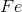) and cobalt (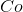), the outermost electrons reside inorbitals. For a free metal, theseorbitals are equal in energy.  However, as soon as ligands (molecules or ions) bind the metal, the metalorbitals split in energy (Figure 1).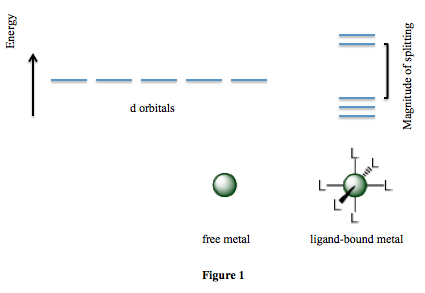Scientists perform a number of experiments to determine how various factors affect the magnitude of splitting. They find that the charge on the metal, the metal's position on the periodic table (whether it resides in Period 4, 5, or 6), and the identity of the ligand are all important factors. The scientists' results are summarized in Table 1. Note that a higher value of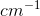indicates larger splitting.

Table 1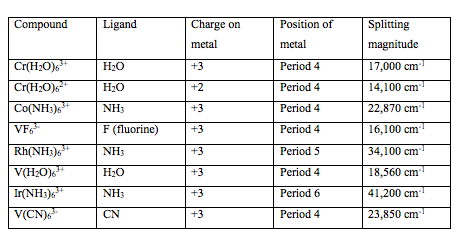Suppose the compound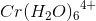had been tested. Based on Table 1, what would a good estimate of the splitting magnitude of this compound be?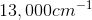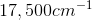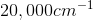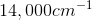Explanation:

As the charge on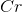increased from +2 to +3, the splitting magnitude increased by about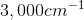. It is reasonable to expect an increase of similar size as the charge ongoes up to +4.

### Example Question #22 : How To Find Data Representation In Physics

An engineer wants to design a prank toy that will be thrown onto the ground. It looks like a superball (bouncing ball), but does not bounce. The engineer has Subtance A, Substance B, and Substance C that he drops from various heights. All of the tests are performed by dropping the substances on the same surface and each height is tested multiple times. The error between experiments is minimal and too small to be seen on the graph. The engineer records the maximum rebound height of each substance at the various drop heights. This data is displayed in the graph below.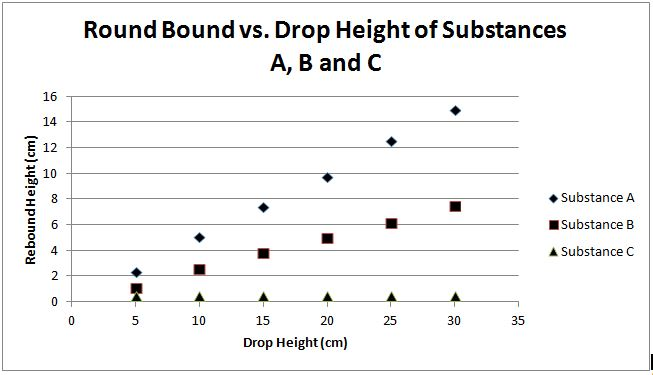If Substance B was dropped from a height of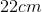, what would be its expected rebound height?

Cannot be determined from the given information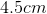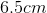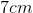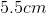Explanation:

One method to solve this problem is to view the graph for Substance B and draw a line up fromon the x-axis and another through the plotted points for Substance B. The intersection of these two lines is aboutand the correct answer.

Alternatively, a process of elimination can be performed. Observation of the figure reveals that Substance B has a linear relationship between plotted points. The data point at the drop height of about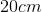has a rebound height of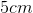. As the drop height increases, so does the rebound height; therefore, the answer cannot bebecause it is too low of a rebound height. At the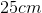drop height, the rebound height is about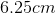. The rebound heights ofandare also eliminated because they are too high. This leaves the answers ofand "Cannot be determined from the given information." The information provided permits the answer to be determined by viewing the plotted points; thus, the correct answer of a rebound height ofis left.

### Example Question #22 : How To Find Data Representation In Physics

An engineer wants to design a prank toy that will be thrown onto the ground. It looks like a superball (bouncing ball), but does not bounce. The engineer has Subtance A, Substance B, and Substance C that he drops from various heights. All of the tests are performed by dropping the substances on the same surface and each height is tested multiple times. The error between experiments is minimal and too small to be seen on the graph. The engineer records the maximum rebound height of each substance at the various drop heights. This data is displayed in the graph below.If the engineer wants to achieve a rebound height of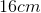from Substance A, approximately what drop height is needed?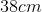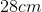None of the choices are correct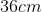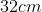Explanation:

As seen in the figure, Substance A possesses a linear relationship between plotted points. Furthermore, there is no information present to suggest that greater drop heights could result in a lower maximum rebound height in future trials of Substance A. In order to answer this question, simply draw a straight line through the data points of Substance A until it intersects themark on the y-axis.  The point parallel to this mark on the x-axis is the correct drop height necessary. The correct answer is### Example Question #23 : How To Find Data Representation In Physics

A researcher is investigating new solar technology. The researcher looks at combinations of solar panels and solar rechargeable batteries that were provided from research labs A, B, C and D. The combinations of solar panels and solar rechargeable batteries are measured for their efficiency, the amount of time it takes to fully charge a battery, and the life of each fully charged battery used to power a home. The experiments were conducted using a UV light. The batteries recharge when the solar panels are exposed to UV light and are depleted of charge when being used for power. The solar panels will not recharge the batteries on cloudy days.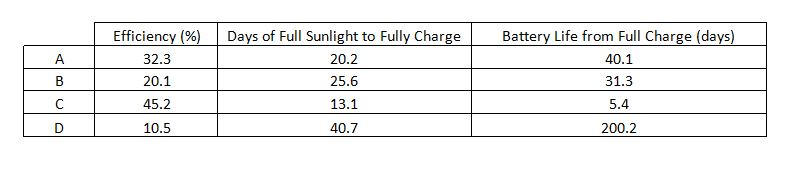Based on the provided data, what relationship can be found between the efficiency of the solar panel and the amount of time it takes to fully charge the battery? Assume that the production differences between batteries and solar panels is negligible across the different labs.

As the efficiency increases, the time it takes to charge the battery decreases

As the efficiency increases, the time it takes to charge the battery increases

There is no relationship between the efficiency and the time it takes to charge the battery

As the efficiency decreases, the time it takes to charge the battery decreases

Cannot be determined

As the efficiency increases, the time it takes to charge the battery decreases

Explanation:

According to the data, it appears that as efficiency increases the time it takes to fully charge the battery decreases. This trend is consistent throughout all the labs; therefore, it is the correct answer. This is true only because the differences between the products from different labs are negligible. In a real-world setting, we would need to observe extraneous variables inherent in each of the different labs and methods of production.

### Example Question #21 : How To Find Data Representation In Physics

Figure 1 shows the electromagnetic spectrum, or the spectrum of energies of light emitted from the sun. The top axis shows each type of electromagnetic wave as a frequency (given in hertz, Hz), where high frequencies correspond with high energy light waves. The bottom axis shows wavelengths (given in meters, m) of each type of light, where short wavelengths correspond with higher energy light waves. There is an inset to show visible light, where higher energy violet light typically has a wavelength around 400nm and 700nm corresponds to lower energy red light. Visible light, as shown in the figure, is typically measured in nanometers (nm), where there are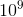nanometers per meter.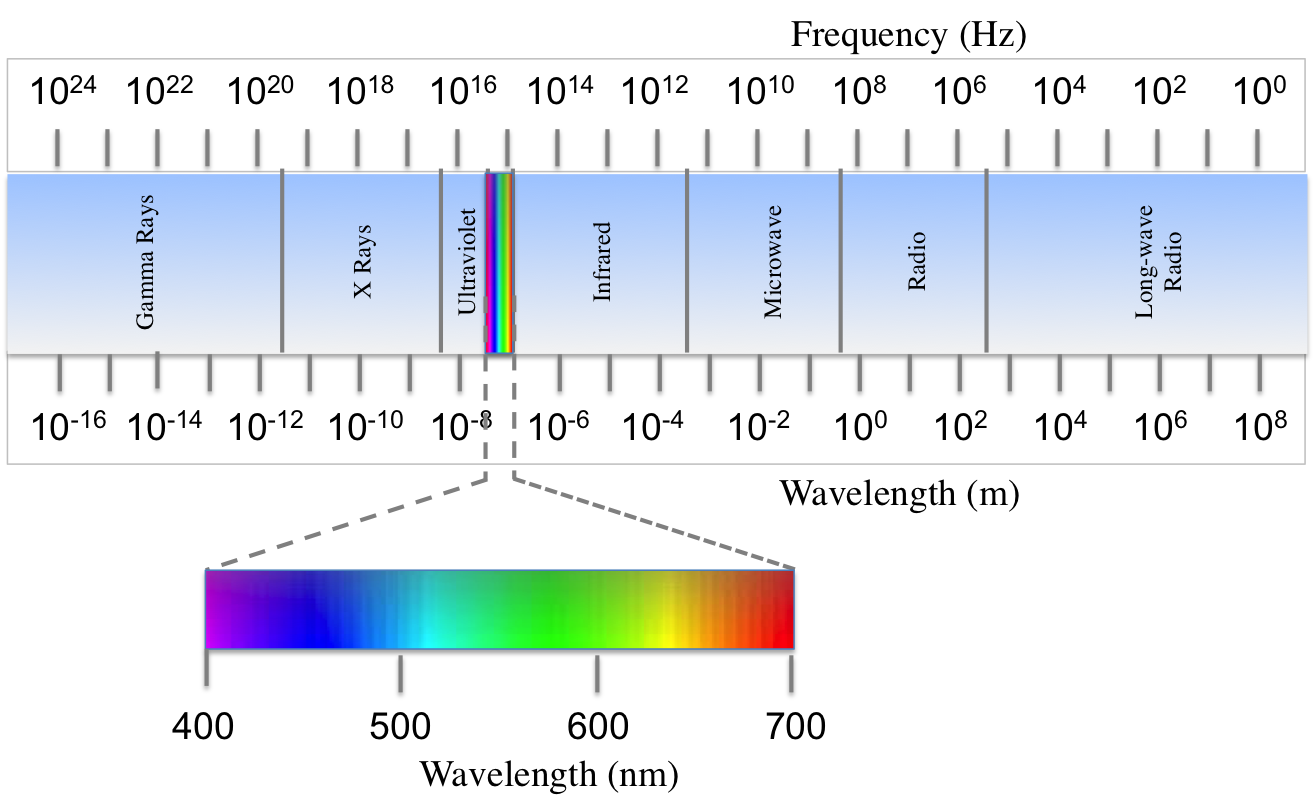Figure 1

A scientist studying the types of electromagnetic radiation emitted from the sun compiled data about the intensity of light at various wavelengths. It was found that less than 1% of radiation from the sun is of wavelengths shorter than 100nm or longer than 2500nm. Intensity of radiation at middle wavelengths was graphed in Figure 2.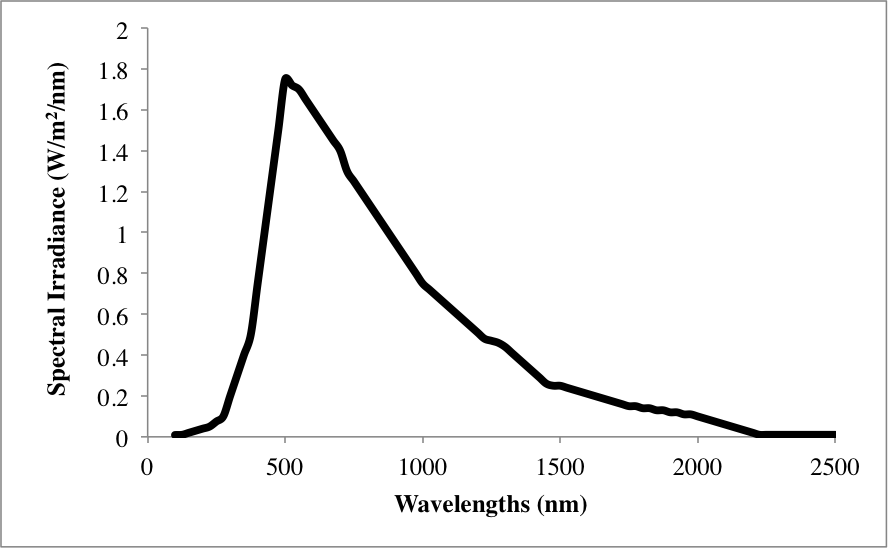Figure 2

Electromagnetic radiation with a frequency of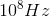has what wavelength, in meters?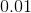Explanation:

According to Figure 1, radiation with a frequency ofcorresponds to radio waves, and has a wavelength of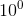. Remember that any number raised to the zeroth power is 1.

### Example Question #24 : How To Find Data Representation In Physics

Figure 1 shows the electromagnetic spectrum, or the spectrum of energies of light emitted from the sun. The top axis shows each type of electromagnetic wave as a frequency (given in hertz, Hz), where high frequencies correspond with high energy light waves. The bottom axis shows wavelengths (given in meters, m) of each type of light, where short wavelengths correspond with higher energy light waves. There is an inset to show visible light, where higher energy violet light typically has a wavelength around 400nm and 700nm corresponds to lower energy red light. Visible light, as shown in the figure, is typically measured in nanometers (nm), where there arenanometers per meter.Figure 1

A scientist studying the types of electromagnetic radiation emitted from the sun compiled data about the intensity of light at various wavelengths. It was found that less than 1% of radiation from the sun is of wavelengths shorter than 100nm or longer than 2500nm. Intensity of radiation at middle wavelengths was graphed in Figure 2.Figure 2

What is the most abundant type of light emitted from the sun?

Infrared light

Red visible light

Green visible light

Ultraviolet light

Green visible light

Explanation:

Figure 2 has a peak at a wavelength roughly longer than 500nm. As the inset in Figure 1 shows that visible light ranges from 400-700nm, we see that it must be some type of visible light. Specifically, wavelengths slightly longer than 500nm appear to be in the green light range.

### Example Question #221 : Physics

Figure 1 shows the electromagnetic spectrum, or the spectrum of energies of light emitted from the sun. The top axis shows each type of electromagnetic wave as a frequency (given in hertz, Hz), where high frequencies correspond with high energy light waves. The bottom axis shows wavelengths (given in meters, m) of each type of light, where short wavelengths correspond with higher energy light waves. There is an inset to show visible light, where higher energy violet light typically has a wavelength around 400nm and 700nm corresponds to lower energy red light. Visible light, as shown in the figure, is typically measured in nanometers (nm), where there arenanometers per meter.Figure 1

A scientist studying the types of electromagnetic radiation emitted from the sun compiled data about the intensity of light at various wavelengths. It was found that less than 1% of radiation from the sun is of wavelengths shorter than 100nm or longer than 2500nm. Intensity of radiation at middle wavelengths was graphed in Figure 2.Figure 2

As you may know, exposure to ultraviolet light is dangerous as it is energetic enough to degrade DNA, leading to cancer. Assuming that all types of radiation that are more energetic than ultraviolet light are also carcinogenic, what of the following types of light likely cause cancer as well?

Gamma rays and x-rays

Visible light and x-rays

Visible light and infrared

Gamma rays and x-rays

Explanation:

According to Figure 1, ultraviolet light has a frequency on the order of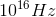and a wavelength on the order of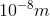. Higher energy waves, which are located to the left of ultraviolet light on the electromagnetic spectrum, should have higher frequencies and smaller wavelengths. This is true of gamma and x-rays.

### Example Question #1341 : Act Science

Figure 1 shows the electromagnetic spectrum, or the spectrum of energies of light emitted from the sun. The top axis shows each type of electromagnetic wave as a frequency (given in hertz, Hz), where high frequencies correspond with high energy light waves. The bottom axis shows wavelengths (given in meters, m) of each type of light, where short wavelengths correspond with higher energy light waves. There is an inset to show visible light, where higher energy violet light typically has a wavelength around 400nm and 700nm corresponds to lower energy red light. Visible light, as shown in the figure, is typically measured in nanometers (nm), where there arenanometers per meter.Figure 1

A scientist studying the types of electromagnetic radiation emitted from the sun compiled data about the intensity of light at various wavelengths. It was found that less than 1% of radiation from the sun is of wavelengths shorter than 100nm or longer than 2500nm. Intensity of radiation at middle wavelengths was graphed in Figure 2.Figure 2

Figure 2 shows an appreciable amount of radiation is produced by the sun with a wavelength of 2000nm. What type of radiation is it, and what is its wavelength in meters?

Microwaves;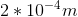Infrared;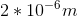Microwaves;Infrared;Infrared;Explanation:

2000nm can be expressed as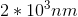. Dimensional analysis shows that: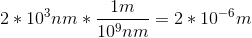Use the given electromagnetic spectrum to match this wavelength to infrared light.

### All ACT Science Resources